# Language, trees, and geometry in neural networks

Part I of a series of expository notes accompanying this paper, by Andy Coenen, Emily Reif, Ann Yuan, Been Kim, Adam Pearce, Fernanda Viégas, and Martin Wattenberg. These notes are designed as an expository walk through some of the main results. Please see the paper for full references and details.

Language is made of discrete structures, yet neural networks operate on continuous data: vectors in high-dimensional space. A successful language-processing network must translate this symbolic information into some kind of geometric representation—but in what form? Word embeddings provide two well-known examples: distance encodes semantic similarity, while certain directions correspond to polarities (e.g. male vs. female).

A recent, fascinating discovery points to an entirely new type of representation. One of the key pieces of linguistic information about a sentence is its syntactic structure. This structure can be represented as a tree whose nodes correspond to words of the sentence. Hewitt and Manning, in A structural probe for finding syntax in word representations, show that several language-processing networks construct geometric copies of such syntax trees. Words are given locations in a high-dimensional space, and (following a certain transformation) Euclidean distance between these locations maps to tree distance.

But an intriguing puzzle accompanies this discovery. The mapping between tree distance and Euclidean distance isn't linear. Instead, Hewitt and Manning found that tree distance corresponds to the square of Euclidean distance. They ask why squaring distance is necessary, and whether there are other possible mappings.

This note provides some potential answers to the puzzle. We show that from a mathematical point of view, squared-distance mappings of trees are particularly natural. Even certain randomized tree embeddings will obey an approximate squared-distance law. Moreover, just knowing the squared-distance relationship allows us to give a simple, explicit description of the overall shape of a tree embedding.

We complement these geometric arguments with analysis and visualizations of real-world embeddings in one network (BERT) and how they systematically differ from their mathematical idealizations. These empirical findings suggest new, quantitative ways to think about representations of syntax in neural networks. (If you're only here for empirical results and visualizations, skip right to that section.)

## Tree embeddings in theory

If you're going to embed a tree into Euclidean space, why not just have tree distance correspond directly to Euclidean distance? One reason is that if the tree has branches, it's impossible to do isometrically.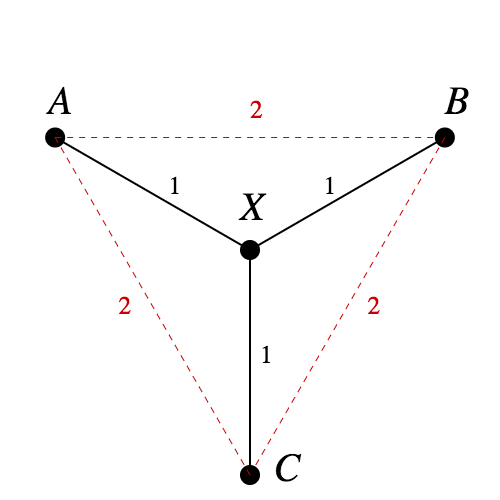Figure 1. You can't isometrically embed this tree in Euclidean space.

In fact, the tree in Figure 1 is one of the standard examples to show that not all metric spaces can be embedded in $\mathbb{R}^n$ isometrically. Since $d(A, B) = d(A, X) + d(X, B)$, in any embedding $A$, $X$, and $B$ will be collinear. The same logic says $A$, $X$, and $C$ will be collinear. But that means $B = C$, a contradiction.

If a tree has any branches at all, it contains a copy of this configuration, and can't be embedded isometrically either.

### Pythagorean embeddings

By contrast, squared-distance embeddings turn out to be much nicer—so nice that we'll give them a name. The reasons behind the name will soon become clear.

Definition: Pythagorean embedding
Let $M$ be a metric space, with metric $d$. We say $f: M \to \mathbb{R}^n$ is a Pythagorean embedding if for all $x, y \in M$, we have $d(x, y) = \|f(x) - f(y)\|^2$.

Does the tree in Figure 1 have a Pythagorean embedding? Yes: as seen in Figure 2, we can assign points to neighboring vertices of a unit cube, and the Pythagorean theorem gives us what we want.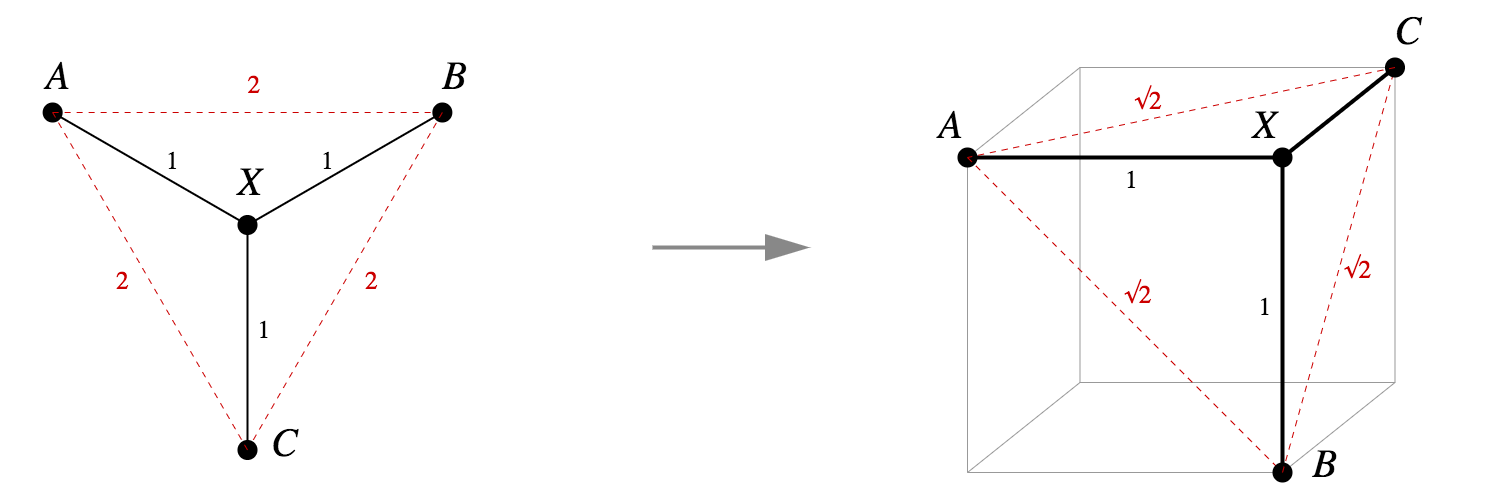Figure 2. A simple Pythagorean embedding into the vertices of a unit cube.

What about other small trees, like a chain of four vertices? That too has a tidy Pythagorean embedding into the vertices of a cube.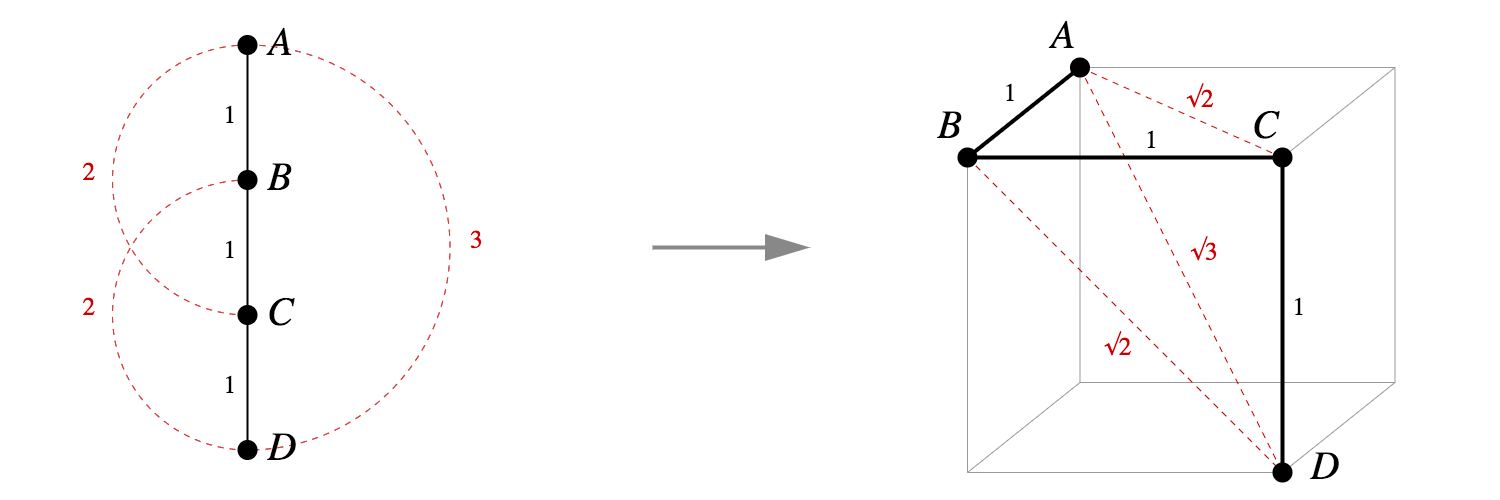Figure 3. A chain of four points also has a Pythagorean embedding into the vertices of a unit cube.

These two examples aren't flukes. It's actually straightforward to write down an explicit Pythagorean embedding for any tree into vertices of a unit hypercube.

Theorem 1.1
Any tree with $n$ nodes has a Pythagorean embedding into $\mathbb{R}^{n-1}$.
Proof.
We've learned that a similar argument to the proof of Theorem 1.1 appears in Hiroshi Maehara's Euclidean embeddings of finite metric spaces.
Let the nodes of the tree $T$ be $t_0, ..., t_{n-1}$, with $t_0$ being the root node. Let $\{e_1, ..., e_{n-1}\}$ be orthogonal unit basis vectors for $\mathbb{R}^{n-1}$. Inductively, define an embedding $\, f: T \rightarrow \mathbb{R}^{n-1}$ by: $$f(t_0) = 0$$ $$f(t_i) = e_i + f(parent(t_i))$$ Given two distinct tree nodes $x$ and $y$, where $m$ is the tree distance $d(x, y)$, it follows that we can move from $f(x)$ to $f(y)$ using $m$ mutually perpendicular unit steps. Thus $$\|f(x) - f(y)\|^2 = m = d(x, y)$$

One way to view this construction is that we've assigned a basis vector to each edge. To figure out the embedding for a node, we walk back to the root and add up all the vectors for the edges we pass. See figure below.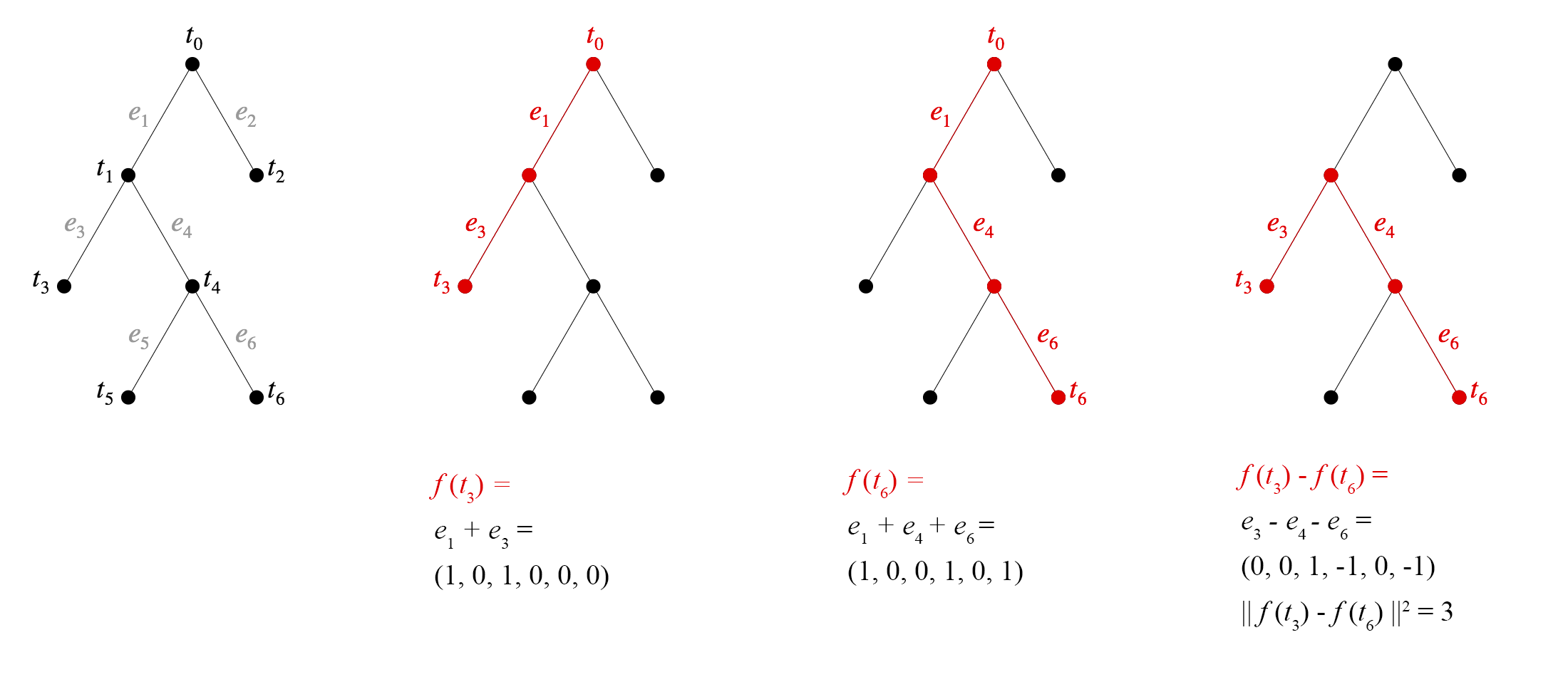Figure 4. Left: Assigning basis vectors to edges. Middle: two example embeddings. Right: Distance squared equals tree distance.
Remarks.

The value of this proof is not just the existence result, but in the explicit geometric construction. Any two Pythagorean embeddings of the same tree are isometric—and related by a rotation or reflection—since distances between all pairs of points are the same in both. So we may speak of the Pythagorean embedding of a tree, and this theorem tells us exactly what it looks like.

Moreover, the embedding in Theorem 1.1 has a clean informal description: at each embedded vertex of the graph, all line segments to neighboring vertices are unit-distance segments, orthogonal to each other and to every other edge segment. If you look at Figures 1 and 2, you'll see they fit this description.

It's also easy to see the specific embedding constructed in the proof is a tree isometry in the $\ell^1$ metric, although this depends strongly on the axis alignment.

We can make a slight generalization of Theorem 1.1. Consider trees where edges have weights, and the distance between two nodes is the sum of weights of the edges on the shortest path between them. In this case, too, we can always create a Pythagorean embedding.

Theorem 1.2.
Any weighted tree with $n$ nodes has a Pythagorean embedding into $\mathbb{R}^{n-1}$.
Proof.
As before, let the nodes of the tree be $t_0, ..., t_{n-1}$, with $t_0$ being the root node. Let $\{e_1, ..., e_{n-1}\}$ be orthogonal unit basis vectors for $\mathbb{R}^{n-1}$. Now let $w_i = d(t_i, parent(t_i))$. Inductively, define an embedding $f$ such that: $$f(t_0) = 0$$ $$f(t_i) = {w_i}^{1/2} e_i + f(parent(t_i))$$
The embedding in Theorem 1.2 no longer lives on the unit hypercube, but rather in a squashed version of it: a rectangular solid whose sides are $\{w_i^{1/2}\}$.
We can index the edges of the tree, with each edge having the same index as the child node on that edge. Let $P$ be the set of indices of edges on the shortest path between $x$ and $y$. Then $$\|f(x) - f(y)\|^2 = \sum_{i \in P}{w_i} = d(x, y)$$

The embedding in Theorem 1.2, despite being axis-aligned, is no longer an isometry with respect to the $\ell^1$ metric. However, if we use vectors $w_i e_i$ rather than ${w_i}^{1/2} e_i$ we can recover an $\ell^1$ isometry.

### Alternative embeddings, and lack thereof

Hewitt and Manning ask whether there might be other effective types of tree embeddings, perhaps based on other powers of the Euclidean metric. We can provide some partial conclusions about such embeddings.

Definition
Let $M$ be a metric space, with metric $d$. We say $f: M \to \mathbb{R}^n$ is a power-$p$ embedding if for all $x, y \in M$, we have $$\|f(x) - f(y)\|^p = d(x, y)$$

For additional expository work on the general question of embeddings into Euclidean space, see this beautiful survey and this useful book chapter.
It turns out that under various names, power-$p$ embeddings of general metric spaces have been studied for many decades. The foundational work is a 1937 paper of Schoenberg. A key result of that paper, phrased in our terminology, is that if a metric space $X$ has a power-$p$ embedding into $\mathbb{R}^n$, then it also has a power-$q$ embedding for any $q > p$. Thus for $p > 2$ there will always be a power-$p$ embedding for any tree. Unlike the case of $p = 2$, we do not know of a simple way to describe the geometry of such an embedding.

On the other hand, it turns out that power-$p$ tree embeddings will not necessarily even exist when $p < 2$.

Theorem 2.
For any $p < 2$, there is a tree which has no power-$p$ embedding.

See our paper for a proof (and an alternative proof may be found here). To summarize the idea, for any given $p < 2$, there is not enough “room” to embed a node with sufficiently many children.

### Randomly branching embeddings are approximately Pythagorean

The Pythagorean embedding property is surprisingly robust, at least in spaces whose dimension is much larger than the size of the tree. (This is the case in our motivating example of language-processing neural networks, for instance.) In the proofs above, instead of using the basis $e_1, \ldots, e_{n-1} \in \mathbb{R}^{n-1}$ we could have chosen $n$ vectors completely at random from a unit Gaussian distribution in $\mathbb{R}^{m}$. If $m \gg n$, with high probability the result would be an approximate Pythagorean embedding.

The reason is that in high dimensions, (1) vectors drawn from a unit Gaussian distribution have length very close to 1 with high probability; and (2) when $m \gg n$, a set of $n$ unit Gaussian vectors will likely be close to mutually orthogonal.

In other words, in a space that is sufficiently high-dimensional, a randomly branching embedding of a tree, where each child is offset from its parent by a random unit Gaussian vector, will be approximately Pythagorean.

This construction could even be done by an iterative process, requiring only “local” information. Initialize with a completely random tree embedding, and in addition pick a special random vector for each vertex; then at each step, move each child node so that it is closer to its parent's location plus the child's special vector. The result will be an approximate Pythagorean embedding.

For more on hyperbolic tree representations, see Hyperbolic Embeddings with a Hopefully Right Amount of Hyperbole, or Nickel & Kiela, Poincaré Embeddings for Learning Hierarchical Representations

The simplicity of Pythagorean embeddings, as well as the fact that they arise from a localized random model, suggests they may be generally useful for representing trees. With the caveat that tree size is controlled by the ambient dimension, they may be a low-tech alternative to approaches based on hyperbolic geometry.

## Tree embeddings in practice

Having described a mathematical idealization of tree embeddings, let's return to the world of neural networks.

BERT background: a Google blog; a nice summary. Many papers analyze these networks, e.g., BERT Rediscovers the Classical NLP Pipeline. Our paper has further references!
Our object of study is the BERT model, a recent, successful model aimed at natural language processing. One reason we're interested in this model is that it performs well for many different tasks, suggesting it is extracting generally useful linguistic features. BERT is an example of a Transformer architecture.

We won't describe the BERT architecture here, but roughly speaking the network takes as input a sequences of words, and across a series of layers produces a series of embeddings for each of these words. Because these embeddings take context into account, they're often referred to as context embeddings.

People have proposed many ways to describe syntactic structure. In dependency grammar each word is a node of the tree.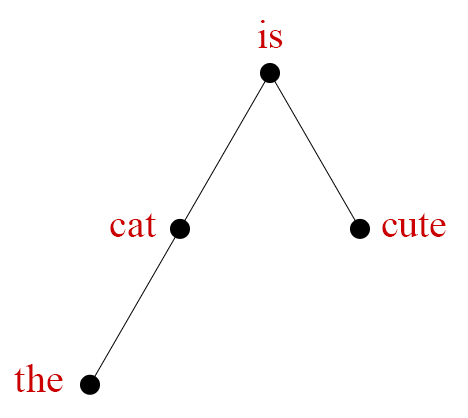Many people have studied these embeddings to see what sort of information they might contain. To recapitulate the introduction, the motivation for our study of tree embeddings was a recent result from Hewitt and Manning. Their paper A structural probe for finding syntax in word representations suggests that context embeddings seem to geometrically encode dependency parse trees.

There is one twist: first you need to transform the context embeddings by a certain matrix $B$, a so-called structural probe. But following that, the square of the Euclidean distance between two words' context embeddings approximates the parse tree distance between the two words.

Here is where the math in the previous section pays off. In our terminology, the context embeddings approximate a Pythagorean embedding of a sentence's dependency parse tree. That means we have a good idea—simply from the squared-distance property and Theorem 1.1—of the overall shape of the tree embedding.

### Visualizing and measuring parse tree representations

Of course, we don't know the shape exactly, because the embedding is only approximately Pythagorean. But the discrepancy between the ideal shape and actual shape is potentially very interesting. Systematic differences between empirical embeddings and their mathematical idealization may provide further clues to how BERT processes language.

PCA produced more readable visualizations than t-SNE or UMAP. Nonlinear methods may do best when points are clustered or distributed on a low-dimensional manifold—pretty much the opposite of vertices of an $n$-cube.
To investigate these differences, we created a visualization tool. Our paper has details, but we'll provide a broad description here. As input, the tool takes a sentence with associated dependency parse tree. The software extracts context embeddings for this sentence from BERT, transformed by the Hewitt and Manning’s “structural probe” matrix, yielding a set of points in 1,024-dimensional space.

We then project these points to two dimensions via PCA. To show the underlying tree structure, we connect pairs of points representing words that have a dependency relation. Figure 5, below, shows the result for a sample sentence and, for comparison, PCA projections for the same data of an exact Pythagorean embedding; randomly branched embeddings; and embeddings where node coordinates are completely random.

Figure 5. a) PCA view of a BERT parse tree embedding. b) an exact Pythagorean embedding. c) different random-branch embeddings. d) different embeddings where node positions are chosen independently at random. Mouse over nodes in any image to compare across embeddings.

The PCA projection is already interesting—there's a definite resemblance between the BERT embedding and the idealization. Figure 5c shows a series of randomly branching embeddings, which also resemble the BERT embedding. As a baseline, Figure 5d shows a series of embeddings where words are placed independently at random.

But we can go one step further, and show how the embedding differs from an idealized model. In Figure 6 below, the color of each edge indicates the difference between Euclidean distance and tree distance. We also connect, with a dotted line, pairs of words without a dependency relation but whose positions (before PCA) were much closer than expected.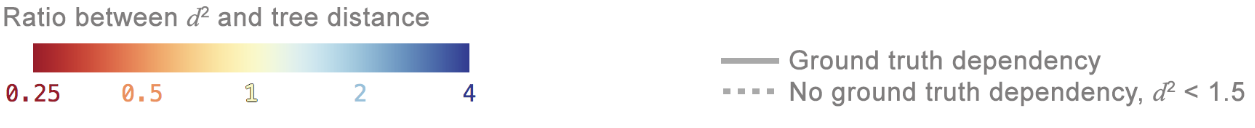Figure 6. Visualizing embeddings of two sentences after applying the Hewitt-Manning probe. Left image in each pair, a traditional parse tree view, but the vertical length of each branch represents embedding distance. Right images: PCA projection of context embeddings, where color shows deviation from expected distance.

The resulting image lets us see both the overall shape of the tree embedding, and fine-grained information on deviation from a true Pythagorean embedding. Figure 5 shows two examples. These are typical cases, illustrating some common themes. In the diagrams, orange dotted lines connect part/of, same/as, and sale/of. This effect is characteristic, with prepositions are embedded unexpectedly close to words they relate to. We also see connections between two nouns showing up as blue, indicating they are farther apart than expected—another common pattern.

Figure 8, at the end of this article, shows additional examples of these visualizations, where you can look for further patterns.

Based on these observations, we decided to make a more systematic study of how different dependency relations might affect embedding distance. One way to answer this question is to consider a large set of sentences and test whether the average distance between pairs of words has any correlation with their syntactic relation.We performed this experiment with a set of Penn Treebank sentences, along with derived parse trees.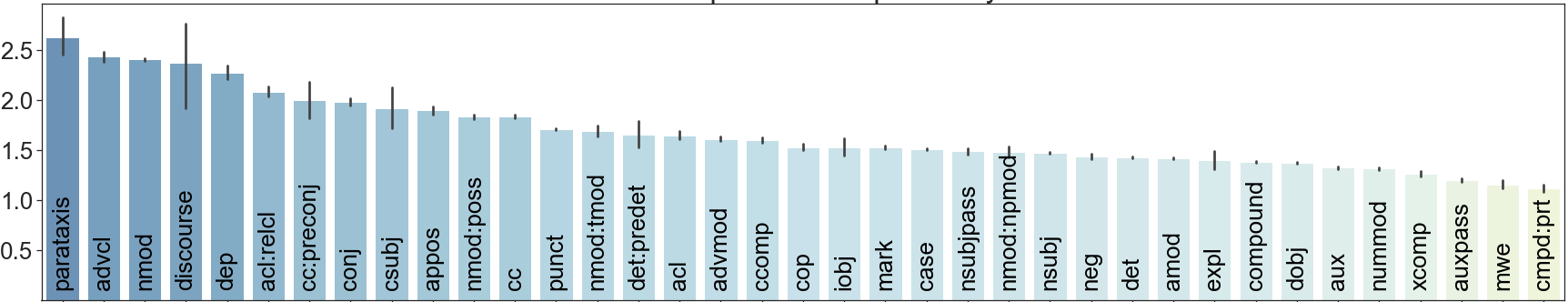Figure 7. The average squared edge length between two words with a given dependency

Figure 7 shows the results of this experiment. It turns out the average embedding distances of each dependency relation vary widely: from around 1.2 (compound : prt, advcl) to 2.5 (mwe, parataxis, auxpass). It is interesting to speculate on what these systematic differences mean. They might be the effects of non-syntactic features, such as word distance within a sentence. Or perhaps BERT’s syntactic representations have additional quantitative aspects beyond plain dependency grammar, using weighted trees.

## Conclusion

Exactly how neural nets represent linguistic information remains mysterious. But we're starting to see enticing clues. The recent work by Hewitt and Manning provides evidence of direct, geometric representations of parse trees. They found an intriguing squared-distance effect, which we argue reflects a mathematically natural type of embedding—and which gives us a surprisingly complete idea of the embedding geometry. At the same time, empirical study of parse tree embeddings in BERT shows that there may be more to the story, with additional quantitative aspects to parse tree representations.

Many thanks to James Wexler for help with this note. And thanks to David Belanger, Tolga Bolukbasi, Dilip Krishnan, D. Sculley, Jasper Snoek, and Ian Tenney for helpful feedback and discussions about this research. For more details, and results related to semantics as well as syntax, please read our full paper! And look for future notes in this series.

Figure 8. Additional parse tree examples; see caption for Figure 5 for details.